# 八个排序的原理、Java 实现以及算法分析## 0. 前言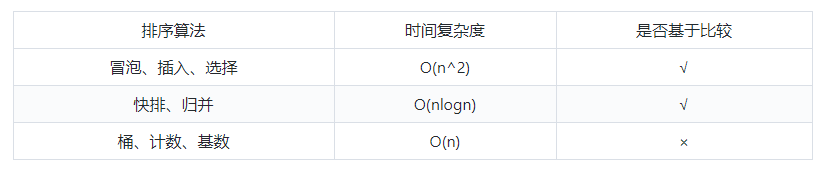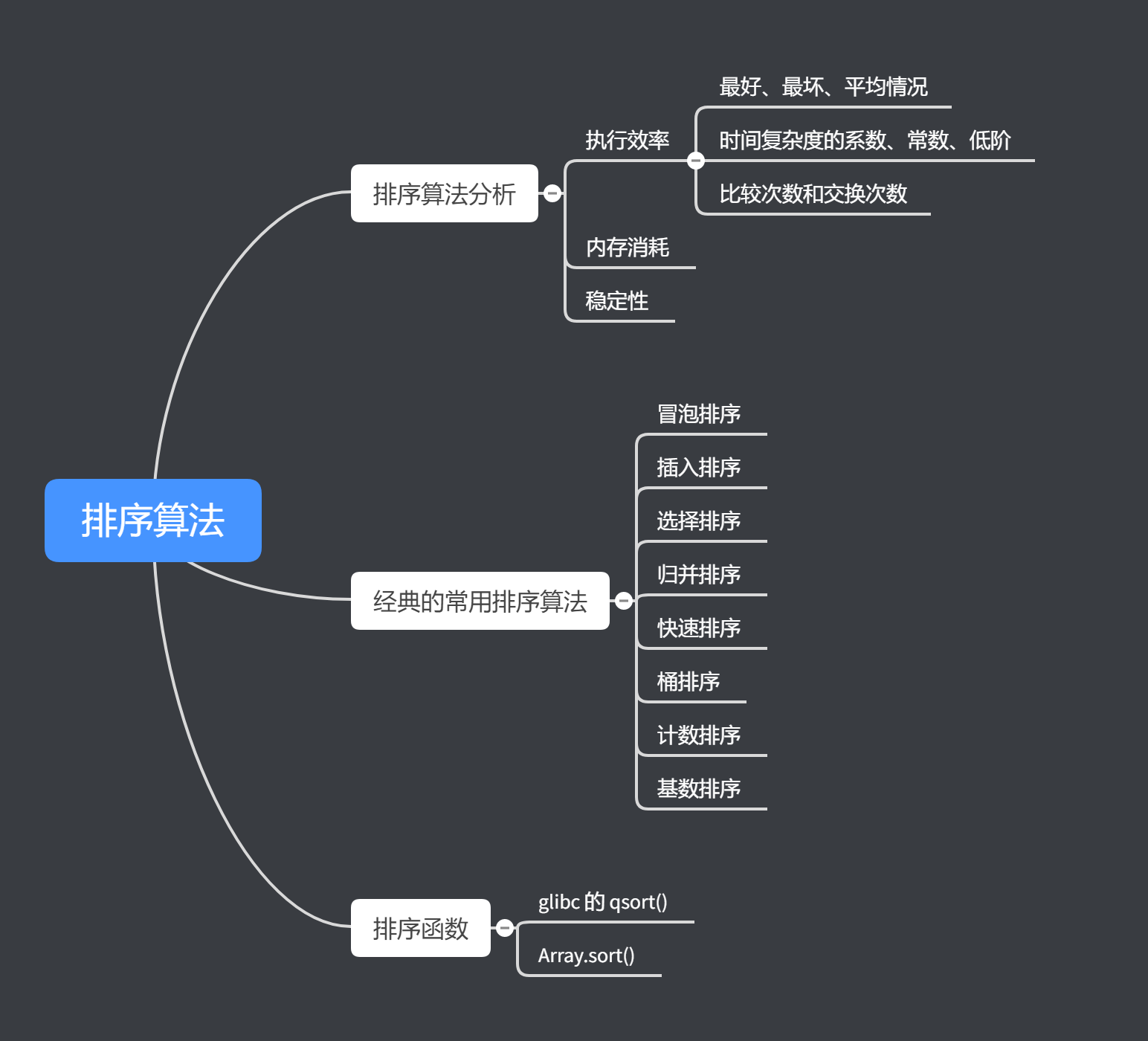## 1. 排序算法分析

### 1.1. 执行效率

• 最好情况、最坏情况、平均情况

• 时间复杂度的系数、常数、低阶

• 比较次数和交换（或移动）次数

## 2. 经典的常用排序算法

### 2.1. 冒泡排序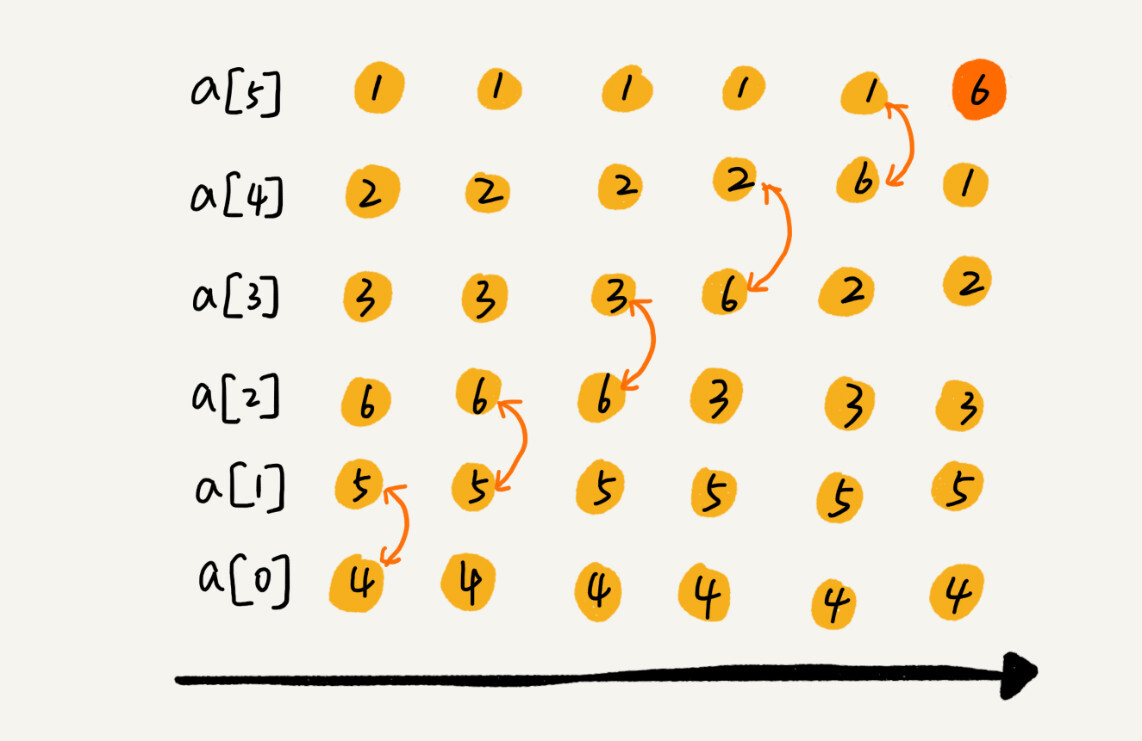﻿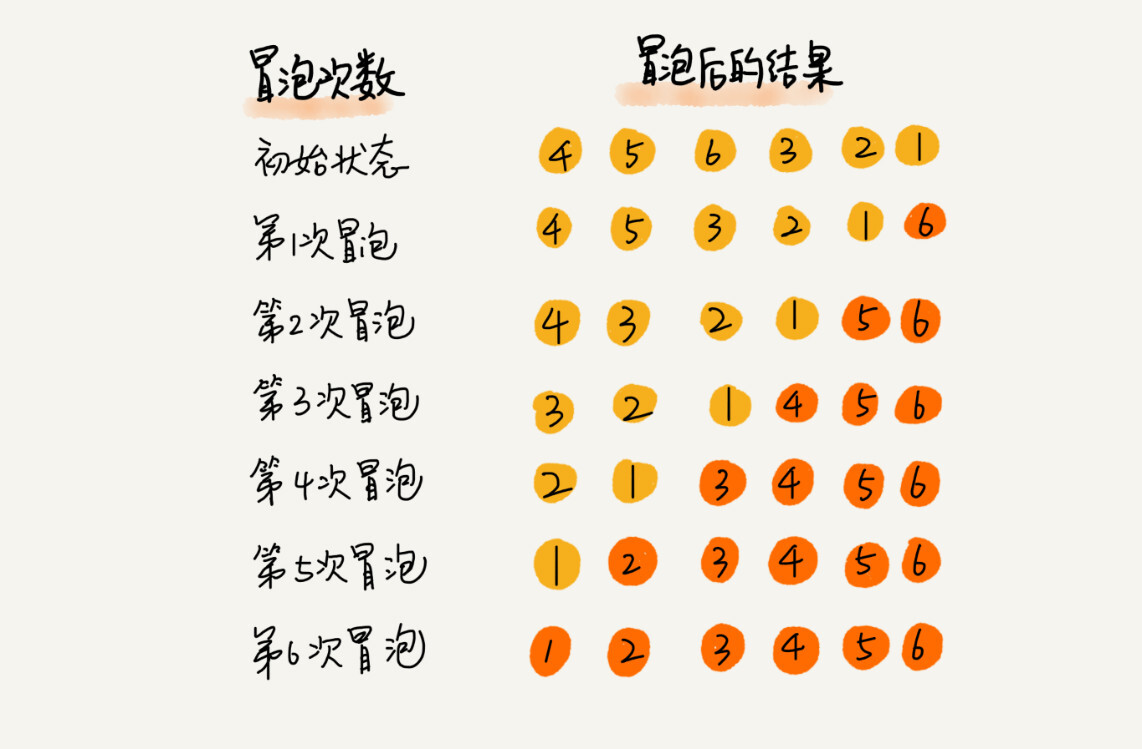﻿

#### 2.1.2. 实现

/** * 冒泡排序： * 以升序为例，就是比较相邻两个数，如果逆序就交换，类似于冒泡； * 一次冒泡确定一个数的位置，因为要确定 n-1 个数，因此需要 n-1  * 次冒泡； * 冒泡排序时，其实相当于把整个待排序序列分为未排序区和已排序区 */public void bubbleSort(int[] arr, int len) {    // len-1 趟    for (int j = 0; j < len-1; j++) {        int sortedFlag = 0;        // 一趟冒泡        for (int i = 0; i < len-1-j; i++) {            if (arr[i] > arr[i+1]) {                int temp = arr[i];                arr[i] = arr[i+1];                arr[i+1] = temp;                sortedFlag = 1;            }        }﻿        // 该趟排序中没有发生，表示已经有序        if (0 == sortedFlag) {            break;        }    }}

#### 2.1.3. 算法分析

• 冒泡排序是原地排序。因为冒泡过程中只涉及到相邻数据的交换，相当于只需要开辟一个内存空间用来完成相邻的数据交换即可。

• 在元素大小相等的时候，不进行交换，那么冒泡排序就是稳定的排序算法。

• 冒泡排序的时间复杂度。

• 当元素已经是排序好了的，那么最好情况的时间复杂度是 O(n)。因为只需要跑一趟，然后发现已经排好序了，那么就可以退出了。

• 当元素正好是倒序排列的，那么需要进行 n-1 趟排序，最坏情况复杂度为 O(n^2)。

• 一般情况下，平均时间复杂度是 O(n^2)。使用有序度和逆序度的方法来求时间复杂度，冒泡排序过程中主要是两个操作：比较和交换。每交换一次，有序度就增加一，因此有序度增加的次数就是交换的次数。又因为有序度需要增加的次数等于逆序度，所以交换的次数其实就等于逆序度

### 2.2. 插入排序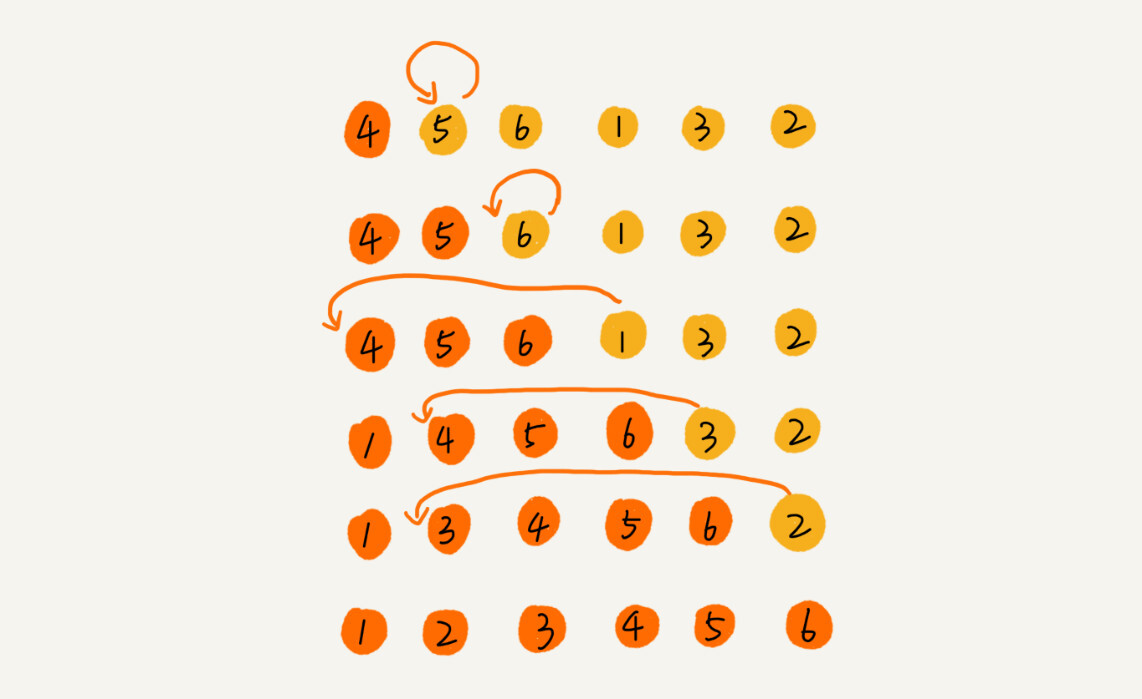﻿

#### 2.2.1. 优化

• 采用希尔排序的方式。

• 使用哨兵机制。比如要排序的数组是[2、1、3、4]，为了使用哨兵机制，首先需要将数组的第 0 位空出来，然后数组元素全都往后移动一格，变成[0、2、1、3、4]。那么数组 0 的位置用来存放要插入的数据，这样一来，判断条件就少了一个，不用再判断 j >= 0 这个条件了，只需要使用 arr[j] > arr 的条件就可以了。因为就算遍历到下标为 0 的位置，由于 0 处这个值跟要插入的值是一样的，所以会退出循环，不会出现越界的问题。

#### 2.2.2. 实现

/** * 插入排序： * 插入排序也相当于把待排序序列分成已排序区和未排序区； * 每趟排序都将从未排序区选择一个元素插入到已排序合适的位置； * 假设第一个元素属于已排序区，那么还需要插入 len-1 趟； */public void insertSort(int[] arr, int len) {    // len-1 趟    for (int i = 1; i < len; i++) {        // 一趟排序        int temp = arr[i];        int j;        for (j = i-1; j >= 0; j--) {            if (arr[j] > temp) {                arr[j+1] = arr[j];            } else {                break;            }        }        arr[j+1] = temp;    }}

#### 2.2.3. 算法分析

• 插入排序是原地算法。因为只需要开辟一个额外的存储空间来临时存储元素。

• 当比较元素时发现元素相等，那么插入到相等元素的后面，此时就是稳定排序。也就是说只有当有序区间中的元素大于要插入的元素时才移到到后面的位置，不大于（小于等于）了的话直接插入。

• 插入排序的时间复杂度。

• 待排序的数据是有序的情况下，不需要搬移任何数据。那么采用从尾到头在已排序区间中查找插入位置的方式，最好时间复杂度是 O(n)。

• 待排序的数据是倒序的情况，需要依次移动 1、2、3、...、n-1 个数据，因此最坏时间复杂度是 O(n^2)。

• 平均时间复杂度是 O(n^2)。因此将一个数据插入到一个有序数组中的平均时间度是 O(n)，那么需要插入 n-1 个数据，因此平均时间复杂度是 O(n^2)

### 2.3. 选择排序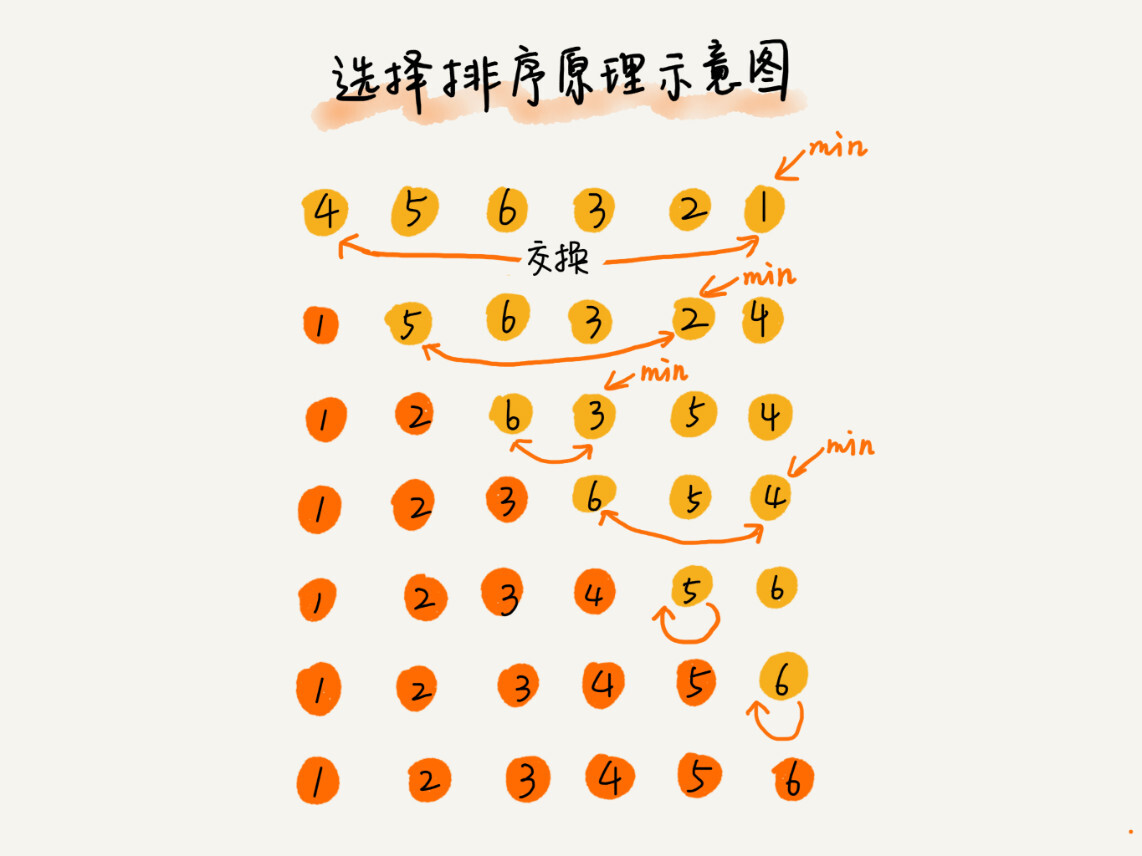﻿

#### 2.3.1. 实现

/** * 选择排序： * 选择排序将待排序序列分成未排序区和已排序区； * 第一趟排序的时候整个待排序序列是未排序区； * 每一趟排序其实就是从未排序区选择一个最值，放到已排序区； * 跑 len-1 趟就好 */public void switchSort(int[] arr, int len) {    // len-1 趟，0-i 为已排序区    for (int i = 0; i < len-1; i++) {        int minIndex = i;        for (int j = i+1; j < len; j++) {            if (arr[j] < arr[minIndex]) {                minIndex = j;            }        }﻿        if (minIndex != i) {            int temp = arr[i];            arr[i] = arr[minIndex];            arr[minIndex] = temp;        }    }}

#### 2.3.2. 算法分析

• 选择排序是原地排序，因为只需要用来存储最小值所处位置的额外空间和交换时所需的额外空间。

• 选择排序不是一个稳定的算法。因为选择排序是从未排序区间中找一个最小值，并且和前面的元素交换位置，这会破坏稳定性。比如 1、5、5、2 这样一组数据中，使用排序算法的话。当找到 2 为 5、5、2 当前未排序区间最小的元素时，2 会与第一个 5 交换位置，那么两个 5 的顺序就变了，就破坏了稳定性。

• 时间复杂度分析。最好、最坏、平均都是 O(n^2)，因为无论待排序数组情况怎么样，就算是已经有序了，都是需要依次遍历完未排序区间，需要比较的次数依次是 n-1、n-2，所以时间复杂度是 O(n^2)。

### 2.4. 归并排序（Merge Sort）

#### 2.4.1. 实现

/** * 归并排序 */public void mergeSort(int[] arr, int len) {    __mergerSort(arr, 0, len-1);}﻿private void __mergerSort(int[] arr, int begin, int end) {    if (begin == end){        return;    }﻿    __mergerSort(arr, begin, (begin+end)/2);    __mergerSort(arr, (begin+end)/2 + 1, end);    merge(arr, begin, end);    return;}﻿private void merge(int[] arr, int begin, int end) {    int[] copyArr = new int[end-begin+1];    System.arraycopy(arr, begin, copyArr, 0, end-begin+1);﻿    int mid = (end - begin + 1)/2;    int i = 0;  // begin - mid 的指针    int j =  mid;   // mid - end 的指针    int count = begin;  // 合并之后数组的指针﻿    while (i <= mid-1 && j <= end - begin) {        arr[count++] = copyArr[i] < copyArr[j] ? copyArr[i++] : copyArr[j++];    }﻿    while (i <= mid-1) {        arr[count++] = copyArr[i++];    }﻿    while (j <= end - begin) {        arr[count++] = copyArr[j++];    }}

#### 2.4.2. 算法分析

• 归并排序可以是稳定的排序算法，只要确保合并时，如果遇到两个相等值的，前半部分那个相等的值是在后半部分那个相等的值的前面即可保证是稳定的排序算法。

• 归并排序的时间复杂度为 O(nlogn)，无论是最好、最坏还是平均情况都一样。

T(n)= 2*T(n/2) + n    = 2*(2*T(n/4)+ n/2)+n = 2^2*T(n/4) + 2*n    = 2^2*(2*T(n/8)+n/4) + 2*n = 2^3*T(n/8) + 3*n    = ....    = 2^k*T(n/2^K) + k*n    = ....    = 2^(log_2^n)*T(1) + log_2^n*n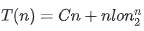• 归并排序并不是原地排序，因为在归并排序的合并函数中，还需要额外的存储空间，这个存储空间是 O(n)。递归过程中，空间复杂度并不能像时间复杂度那样累加。因为在每次递归下去的过程中，虽然合并操作都会申请额外的内存空间，但是合并之后，这些申请的内存空间就会被释放掉。因此其实主要考虑最大问题合并时所需的空间复杂度即可，该空间复杂度为 O(n)。

### 2.5. 快速排序（Quick Sort）

#### 2.5.1. 实现

• 首先说明这种方法不可取。在不考虑空间消耗的情况下，分区操作可以非常简单。使用两个临时数组 X 和 Y，遍历 begin 到 end 之间的数据，将小于 pivot 的数据都放到数组 X 中，将大于 pivot 的数据都放到数组 Y 中，最后将数组 X 拷贝到原数组中，然后再放入 pivot，最后再放入数组 Y。但是采用这种方式之后，快排就不是原地排序算法了，因此可以采用以下两种方法在原数组的基础之上完成分区操作。

• 第一种方法还是使用两个指针：i 和 j，i 和 j 一开始都放置在 begin 初。之后 j 指针开始遍历，如果 j 指针所指的元素小于等于 pivot，那么则将 j 指针的元素放到 i 指针的处，i 指针的元素放置于 j 处，然后 i 后移，j 后移。如果 j 指针所指的元素大于 pivot 那么 j 后移即可。首先个人觉得其实整个数组被分成三个区域：0-i-1 的为小于等于 pivot 的区域，i-j-1 为大于 pivot 的区域，j 之后的区域是未排序的区域。

• 第二种方法还是使用两个指针：i 和 j，i 从 begin 处开始，j 从 end 处开始。首先 j 从 end 开始往前遍历，当遇到小于 pivot 的时候停下来，然后此时 i 从 begin 开始往后遍历，当遇到大于 pivot 的时候停下来，此时交换 i 和 j 处的元素。之后 j 继续移动，重复上述过程，直至 i >= j。

/** * 快速排序 */public void quickSort(int[] arr, int len) {    __quickSort(arr, 0, len-1);}﻿// 注意边界条件private void __quickSort(int[] arr, int begin, int end) {    if (begin >= end) {        return;    }﻿    // 一定要是 p-1！    int p = partition(arr, begin, end); // 先进行大致排序，并获取区分点    __quickSort(arr, begin, p-1);    __quickSort(arr, p+1, end);}﻿private int partition(int[] arr, int begin, int end) {    int pValue = arr[end];﻿    // 整两个指针，两个指针都从头开始    // begin --- i-1（含 i-1）： 小于 pValue 的区    // i --- j-1（含 j-1）：大于 pValue 的区    // j --- end：未排序区    int i = begin;    int j = begin;    while (j <= end) {        if (arr[j] <= pValue) {            int temp = arr[j];            arr[j] = arr[i];            arr[i] = temp;            i++;            j++;        } else {            j++;        }    }﻿    return i-1;}

#### 2.5.2. 优化

• 由于分区点很重要（为什么重要见算法分析），因此可以想方法寻找一个好的分区点来使得被分区点分开的两个分区中，数据的数量差不多。下面介绍两种比较常见的算法：

• 三数取中法。就是从区间的首、尾、中间分别取出一个数，然后对比大小，取这 3 个数的中间值作为分区点。但是，如果排序的数组比较大，那“三数取中”可能不够了，可能就要“五数取中”或者“十数取中”，也就是间隔某个固定的长度，取数据进行比较，然后选择中间值最为分区点。

• 随机法。随机法就是从排序的区间中，随机选择一个元素作为分区点。随机法不能保证每次分区点都是比较好的，但是从概率的角度来看，也不太可能出现每次分区点都很差的情况。所以平均情况下，随机法取分区点还是比较好的。

• 递归可能会栈溢出，最好的方式是使用非递归的方式；

#### 2.5.3. 算法分析

• 快排不是一个稳定的排序算法。因为分区的过程涉及到交换操作，原本在前面的元素可能会被交换到后面去。比如 6、8、7、6、3、5、9、4 这个数组中。在经过第一次分区操作之后，两个 6 的顺序就会发生改变。

• 快排是一种原地的排序算法。

• 快排的最坏时间复杂度是 O(n^2)，最好时间复杂度是O(nlogn)，平均时间复杂度是 O(nlogn)。

• 假设每次分区操作都可以把数组分成大小接近相等的两个小区间，那么快排的时间复杂度和归并排序一样，都是 O(nlogn)。

• 但是分区操作不一定都能把数组分成大小接近相等的两个小区间。极端情况如数组中的数组已经有序了，如果还是取最后一个元素作为分割点，左边区间是 n-1 个数，右边区间没有任何数。此时， T(n)=T(n-1)+n，最终时间复杂度退化为 O(n^2)。大部分情况下，采用递归树的方法可得到时间复杂度是 O(nlogn)。由于极端情况是少数，因此平均时间复杂度是 O(nlogn)。

### 2.6. 桶排序

• 先确定要排序的数据的范围；

• 然后根据范围将数据分到桶中（可以选择桶的数量固定，也可以选择桶的大小固定）；

• 之后对每个桶进行排序；

• 之后将桶中的数据进行合并；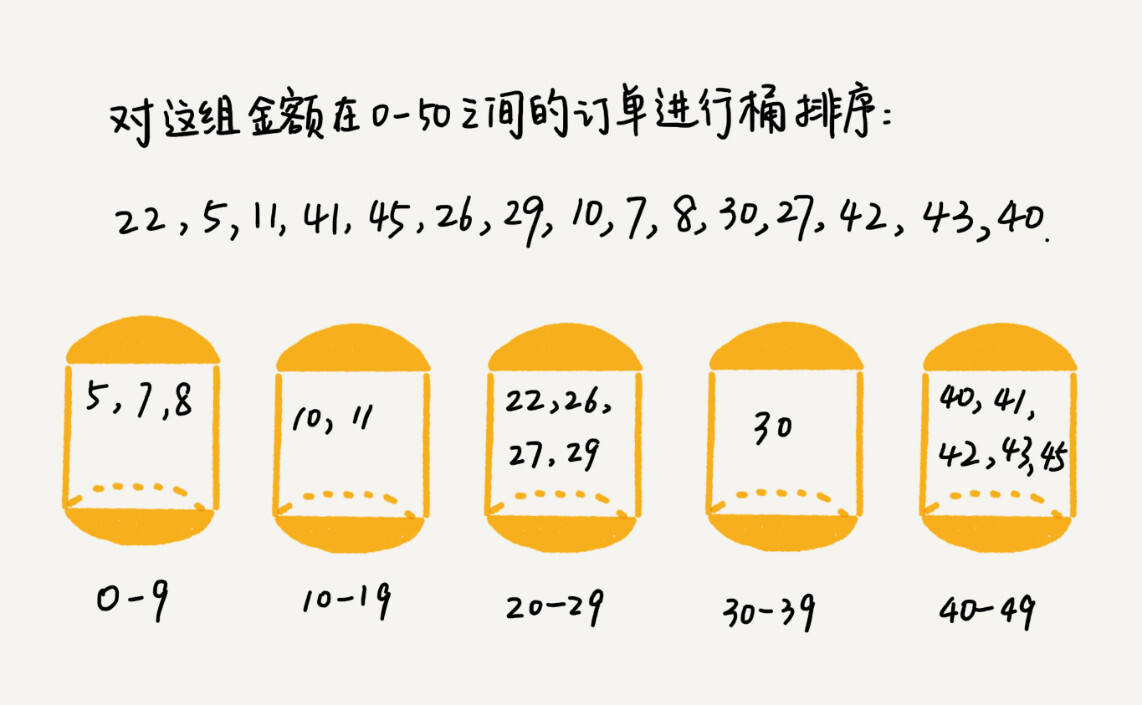#### 2.6.1. 实现

public void buckerSort(int[] arr, int len, int bucketCount) {﻿    // 确定数据的范围    int minVal = arr;    int maxVal = arr;    for (int i = 1; i < len; ++i) {        if (arr[i] < minVal) {            minVal = arr[i];        } else if (arr[i] > maxVal){            maxVal = arr[i];        }    }﻿    // 确认每个桶的所表示的范围    bucketCount =  (maxVal - minVal + 1) < bucketCount ? (maxVal - minVal + 1) : bucketCount;    int bucketSize = (maxVal - minVal + 1) / bucketCount;    bucketCount = (maxVal -  minVal + 1) % bucketCount == 0 ? bucketCount : bucketCount + 1;﻿    int[][] buckets = new int[bucketCount][bucketSize];    int[] indexArr = new int[bucketCount];  // 数组位置记录﻿    // 将数据依次放入桶中    for (int i = 0; i < len; i++) {        int bucketIndex = (arr[i] - minVal) / bucketSize;        if (indexArr[bucketIndex] == buckets[bucketIndex].length) {            expandCapacity(buckets, bucketIndex);        }        buckets[bucketIndex][indexArr[bucketIndex]++] = arr[i];     }﻿    // 桶内排序    for (int i = 0; i < bucketCount; ++i) {        if (indexArr[i] != 0) {            quickSort(buckets[i], 0, indexArr[i] - 1);        }    }﻿    // 桶内数据依次取出    int index = 0;    for (int i = 0; i < bucketCount; ++i) {        for (int j = 0; j < indexArr[i]; ++j) {            arr[index++] = buckets[i][j];        }    }﻿    // 打印    for (int i = 0; i < len; ++i) {        System.out.print(arr[i] + " ");    }    System.out.println();}﻿// 对数组进行扩容public void expandCapacity(int[][] buckets, int bucketIndex) {    int[] newArr = new int[buckets[bucketIndex].length * 2];    System.arraycopy(buckets[bucketIndex], 0, newArr, 0, buckets[bucketIndex].length);    buckets[bucketIndex] = newArr;}

#### 2.6.2. 算法分析

• 最好时间复杂度为 O(n)，最坏时间复杂度为 O(nlogn)，平均时间复杂度为 O(n)。

• 非原地算法

• 桶排序的稳定与否，主要看两块：1.将数据放入桶中的时候是否按照顺序放入；2.桶内采用的排序算法。所以将数据放入桶中是按照顺序的，并且桶内也采用稳定的排序算法的话，那么整个桶排序则是稳定的。既然能稳定的话，那么一般算稳定的。

#### 2.6.3. 总结

• 桶排序对要排序的数据的要求是非常苛刻的。

• 首先，要排序的数据需要很容易被划分到 m 个桶。并且，桶与桶之间有着天然的大小顺序，这样子每个桶内的数据都排序完之后，桶与桶之间的数据不需要再进行排序；

• 其次，数据在各个桶中的分布是比较均匀的。如果数据经过桶的划分之后，每个桶的数据很不平均，比如一个桶中包含了所有数据，那么桶排序就退化为 O(nlogn) 的排序算法了。

• 桶排序适合应用在外部排序中。比如要排序的数据有 10 GB 的订单数据，但是内存只有几百 MB，无法一次性把 10GB 的数据全都加载到内存中。这个时候，就可以先扫描 10GB 的订单数据，然后确定一下订单数据的所处的范围，比如订单的范围位于 1~10 万元之间，那么可以将所有的数据划分到 100 个桶里。再依次扫描 10GB 的订单数据，把 1~1000 元之内的订单存放到第一个桶中，1001~2000 元之内的订单数据存放到第二个桶中，每个桶对应一个文件，文件的命名按照金额范围的大小顺序编号如 00、01，即第一个桶的数据输出到文件 00 中。

﻿

### 2.7. 计数排序

• 首先使用大小为 6 的数组 C 来存储每个值的个数，下标对应具体值。从而得到，C 的情况为：2、0、2、3、0、1。

• 那么，值为 3 分的数据个数有 3 个，小于 3 分的数据个数有 4 个，所以值为 3 的数据在有序数组 R 中所处的位置应该是 4、5、6。为了快速计算出位置，对 C 这个数组进行变化，C[k] 里存储小于等于值 k 的数据个数。变化之后的数组为 2、2、4、7、7、8。

• 之后我们从后往前依次扫描数据 A（从后往前是为了稳定），比如扫描到 3 的时候，从数据 C 中取出下标为 3 的值，是7（也就说到目前为止，包含自己在内，值小于等于 3 的数据个数有 7 个），那么 3 就是数组 R 中第 7 个元素，也就是下标为 6。当然 3 放入到数组 R 中后，C 要减 1，变成 6，表示此时未排序的数据中小于等于 3 的数据个数有 6 个。

• 以此类推，当扫描到第 2 个值为 3 的数据的时候，就会将这个数据放入到 R 中下标为 5 的位置。当扫描完整个数组 A 后，数组 R 内的数据就是按照值从小到大的有序排列了。

#### 2.7.1. 实现

/** * 计数排序，暂时只能处理整数（包括整数和负数） * @param arr * @param len */public void countingSort(int[] arr, int len) {    // 确定范围    int minVal = arr;    int maxVal = arr;    for (int i = 1; i < len; ++i) {        if (maxVal < arr[i]) {            maxVal = arr[i];        } else if (arr[i] < minVal) {            minVal = arr[i];        }    }﻿    // 对数据进行处理    for (int i = 0; i < len; ++i) {        arr[i] = arr[i] - minVal;    }    maxVal = maxVal - minVal;﻿    // 遍历数据数组，求得计数数组的个数    int[] count = new int[maxVal + 1];    for (int i = 0; i < len; ++i) {        count[arr[i]] ++;    }    printAll(count, maxVal + 1);﻿    // 对计数数组进行优化    for (int i = 1; i < maxVal + 1; ++i) {        count[i] = count[i - 1] + count[i];    }    printAll(count, maxVal + 1);﻿    // 进行排序，从后往前遍历（为了稳定）    int[] sort = new int[len];    for (int i = len - 1; i >= 0; --i) {        sort[count[arr[i]] - 1] = arr[i] + minVal;        count[arr[i]]--;    }    printAll(sort, len);}

• 非原地算法

• 稳定算法

• 时间复杂度

#### 2.7.3. 总结

• 计数排序只能用在数据范围不大的场景中，如果数据范围 k 比要排序的数据 n 大很多，就不适合用计数排序了。

• 计数排序只能直接对非负整数进行排序，如果要排序的数据是其他类型的，需要在不改变相对大小的情况下，转化为非负整数。比如当要排序的数是精确到小数点后一位时，就需要将所有的数据的值都先乘以 10，转换为整数。再比如排序的数据中有负数时，数据的范围是[-1000,1000]，那么就需要先将每个数据加上 1000，转换为非负整数。

### 2.8. 基数排序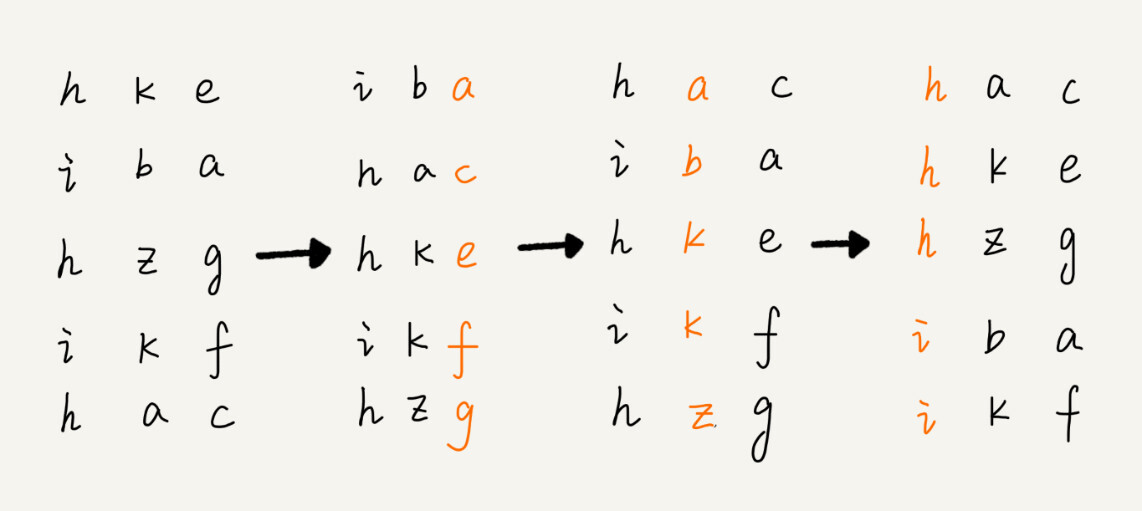﻿

#### 2.8.1. 实现

/** * 基数排序 * @param arr * @param len */public void radixSort(int[] arr, int len, int bitCount) {    int exp = 1;    for (int i = 0; i < bitCount; ++i) {        countingSort(arr, len, exp);        exp = exp * 10;    }}﻿public int getBit(int value, int exp) {    return (value / exp) % 10;}/**     * 计数排序，暂时只能处理整数（包括整数和负数）     * @param arr     * @param len     */public void countingSort(int[] arr, int len, int exp) {﻿    // 确定范围    int maxVal = getBit(arr, exp);    for (int i = 1; i < len; ++i) {        if (maxVal < getBit(arr[i], exp)) {            maxVal = getBit(arr[i], exp);        }    }﻿    // 遍历数据数组，求得计数数组的个数    int[] count = new int[maxVal + 1];    for (int i = 0; i < len; ++i) {        count[getBit(arr[i], exp)] ++;    }﻿    // 对计数数组进行优化    for (int i = 1; i < maxVal + 1; ++i) {        count[i] = count[i - 1] + count[i];    }﻿    // 进行排序，从后往前遍历（为了稳定）    int[] sort = new int[len];    for (int i = len - 1; i >= 0; --i) {        sort[count[getBit(arr[i], exp)] - 1] = arr[i];        count[getBit(arr[i], exp)]--;    }    System.arraycopy(sort, 0, arr, 0, len);    printAll(sort, len);}

#### 2.8.2. 算法分析

• 非原地算法

• 稳定算法

• 时间复杂度是 O(kn)，k 是数组的位数

#### 2.8.3. 总结

• 基数排序的一个要求是排序的数据要是等长的。当不等长时候可以在前面或者后面补 0，比如字符串排序的话，就可以在后面补 0，因为 ASCII 码中所有的字母都大于 “0”，所以补 “0” 不会影响到原有的大小排序。

• 基数排序的另一个要求就是数据可以分割出独立的 “位” 来比较，而且位之间存在递进关系：如果 a 数据的高位比 b 数据大，那么剩下的低位就不用比较了。

• 除此之外，每一个位的数据范围不能太大，要能用线性排序算法来排序，否则，基数排序时间复杂度无法达到 O(n)。

## 3. 排序函数

• glibc 的 qsort() 排序函数。qsort() 会优先使用归并排序算法。当排序的数据量很大时，会使用快速排序。使用排序算法的时候也会进行优化，如使用 “三数取中法”、在堆上手动实现一个栈来模拟递归来解决。在快排的过程中，如果排序的区间的元素个数小于等于 4 时，则使用插入排序。而且在插入排序中还用到了哨兵机制，减少了一次判断。

• Array.sort() 排序函数，使用 TimSort 算法。TimSort 算法是一种归并算法和插入排序算法混合的排序算法。基本工作过程就是：

• 扫描数组，确定其中的单调上升段和单调下降段，将严格下降段反转；

• 定义最小基本片段长度，长度不满足的单调片段通过插入排序的方式形成满足长度的单调片段（就是长度大于等于所要求的最小基本片段长度）

• 反复归并一些相邻片段，过程中避免归并长度相差很大的片段，直至整个排序完成。

## 4. 附加知识

### 4.1. 有序度、逆序度

如果 i < j，那么 a[i] < a[j]

如果 i < j，那么 a[i] > a[j]

逆序度 = 满有序度 - 有序度

## 5. 总结

• 冒泡排序、选择排序可能就停留在理论的层面，实际开发应用中不多，但是插入排序还是挺有用的，有些排序算法优化的时候就会用到插入排序，比如在排序数据量小的时候会先选择插入排序。

• 冒泡、选择、插入三者的时间复杂度一般都是按 n^2 来算。并且这三者都有一个共同特点，那就是都会将排序数列分成已排序和未排序两部分。外层循环一次，其实是让有序部分增加一个，因此外层循环相当于对有序部分和未排序部分进行分割。而外层循环次数就是待排序的数据的个数；内层循环则主要负责处理未排序部分的元素。

• 快排的分区过程和分区思想其实特别好用，在解决很多非排序的问题上都会遇到。比如如何在 O(n) 的时间复杂度内查找一个 k 最值的问题（还用到分治，更多是分区这种方式）；比如将一串字符串划分成字母和数字两部分（其实就是分区，所以需要注意分区过程的应用）。以后看到类似分区什么的，可以想想快排分区过程的操作。

• 快排和归并使用都是分治的思想，都可使用递归的方式实现。只是归并是从下往上的处理过程，是先进行子问题处理，然后再合并；而快排是从上往下的处理过程，是先进行分区，而后再进行子问题处理。

• 桶排序、计数排序、基数排序的时间复杂度是线性的，所以这类排序算法叫做线性排序。之所以这能做到线性排序，主要是因为这三种算法都不是基于比较的排序算法，不涉及到元素之间的比较操作。但是这三种算法对排序的数据要求很苛刻。如果数据特征比较符合这些排序算法的要求，这些算法的复杂度可以达到 O(n)。

• 桶排序、计数排序针对范围不大的数据是可行的，它们的基本思想都是将数据划分为不同的桶来实现排序。

• 各种算法比较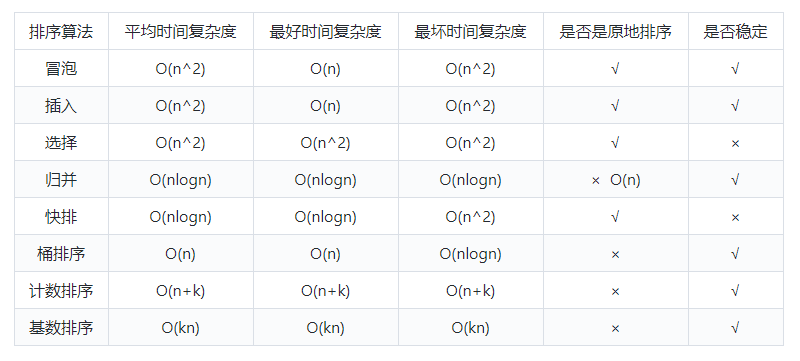﻿

## 巨人的肩膀

1. 极客时间，《数据结构与算法之美》，王争

2. 《算法图解》

﻿## 评论